# Airborne SAR System Design

This example shows how to design a synthetic aperture radar (SAR) sensor operating in the X-band and calculate the sensor parameters. SAR uses the motion of the radar antenna over a target region to provide an image of the target region. A synthetic aperture is created when the SAR platform travels over the target region while pulses are transmitted and received from the radar antenna.

This example focuses on designing an SAR sensor to meet a set of performance parameters. It outlines the steps to translate performance specifications, such as the azimuth resolution and the probability of detection, into SAR system parameters, such as the antenna dimension and the transmit power. It models design parameters for stripmap and spotlight modes of operation. Compared to the stripmap operation, spotlight mode can provide a better resolution, and a stronger signal from the scene at the cost of reduced scene size or area imaging rate. The example also models the parameters of the azimuth chirp signal.

The diagram below classifies the various system and performance parameters. This example covers the functions for selecting system parameters to meet performance parameters.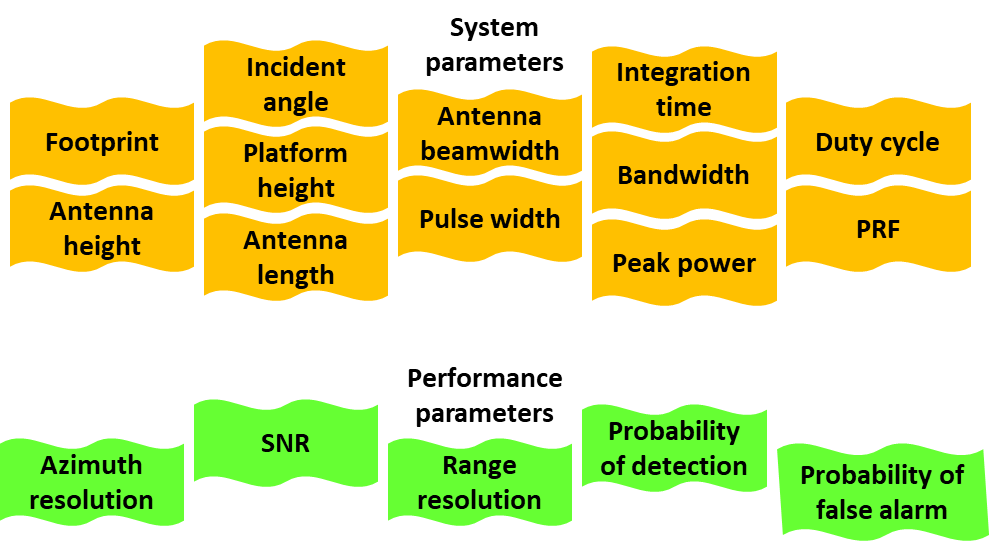### Design Specifications

The goal of this airborne SAR system is to provide an image of the target region at a distance up to 10 km from the airborne platform with a range and azimuth resolution of 1 m. The platform is operating at an altitude of 5 km and moving at a velocity of 100 m/s. The desired performance indices are the probability of detection (Pd) and probability of false alarm (Pfa). The Pd value must be 0.9 or greater. The Pfa value must be less than 1e-6.

```slantrngres = 1; % Required slant range resolution (m) azres = 1; % Required azimuth resolution (m) maxrng = 10e3; % Maximum unambiguous slant range (m) pd = 0.9; % Probability of detection pfa = 1e-6; % Probability of false alarm v = 100; % Velocity (m/s) h = 5000; % Radar altitude (m)```

### Airborne SAR System Design

System parameters like length of synthetic aperture, integration time, coverage rate, beamwidth for stripmap as well as spotlight modes, and signal bandwidth are key parameters that define the operational capability of a SAR system. These parameters ensure that the SAR system covers the region of interest for the calculated integration time with a wide beam. The calculated signal bandwidth meets the desired range resolution.

Signal Configuration

To calculate the SAR system parameters, you must first know the wavelength of the propagating signal, which is inversely related is to the operating frequency of the system. For this example, set the operating frequency to 10 GHz which is typical airborne SAR systems.

Use the `freq2wavelen` function to calculate the wavelength of the propagating signal.

```freq = 10e9; % Radar frequency within X-band (Hz) lambda = freq2wavelen(freq) % Wavelength (m)```
```lambda = 0.0300 ```

The signal bandwidth maps to the slant range resolution of SAR and the slant range resolution is the factor needed to distinguish two targets separated by a distance. The slant range resolution gives you the minimum range difference needed to distinguish two targets. Use the `rangeres2bw` function to calculate the signal bandwidth, which is determined by the slant range resolution.

`pulse_bw = rangeres2bw(slantrngres) % Pulse bandwidth (Hz)`
```pulse_bw = 149896229 ```

Stripmap SAR Mode

Stripmap SAR mode assumes a fixed pointing direction of the radar antenna relative to the direction of motion of platform. The antenna in this example points to the broadside direction.

Antenna Orientation

The depression angle is often used to define the antenna pointing direction in elevation. This example assumes that the earth is flat so that the depression angle is the same as the grazing angle.

Use the `grazingang` function to calculate the grazing angle from the line-of-sight range.

`grazang = grazingang(h,maxrng,'Flat') % Grazing angle (in degrees)`
```grazang = 30.0000 ```

Antenna Azimuth Dimension

Next, use the `sarlen` and `sarazres` functions to analyze and calculate the synthetic aperture length and its azimuth resolution for selecting the antenna azimuth dimension. Plot the synthetic length as a function of cross-range resolution. Plot the antenna azimuth dimension as a function of synthetic length.

```dazv = [1 1.5 2 2.5 3]; % Antenna azimuth dimensions (m) striplenv = zeros(1,numel(dazv)); stripazresv = zeros(1,numel(dazv)); for i=1:numel(dazv) striplenv(i) = sarlen(maxrng,lambda,dazv(i)); stripazresv(i) = sarazres(maxrng,lambda,striplenv(i)); end helperPlotStripmapMode(stripazresv,striplenv,dazv,azres)```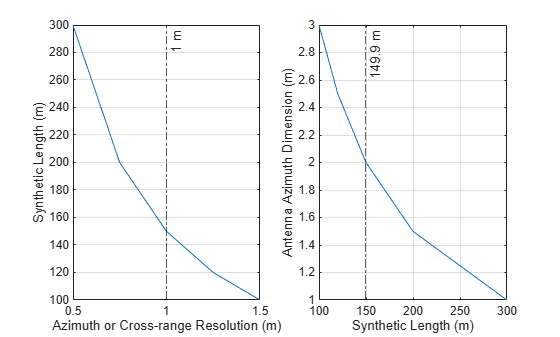The figures show that a synthetic aperture length of 149.9 m for stripmap mode is a good value to meet a required azimuth resolution of 1 m. The smallest antenna azimuth dimension you can use for stripmap mode in this scenario is 2 m. Decrease the antenna azimuth dimension to obtain a better azimuth resolution than 1 m for stripmap mode.

Set the synthetic aperture length to 149.9 m for stripmap mode and the antenna azimuth dimension of 2 m.

`daz = 2`
```daz = 2 ```
`striplen = 149.9`
```striplen = 149.9000 ```

Antenna Elevation Dimension

Next, determine the antenna elevation dimension based on the required swath length. For this example, assume that the required swath length is 2.4 km.

Use the `aperture2swath` function to analyze the swath length for selecting an antenna elevation dimension.

```rngswath = 2400; delv = [0.15 0.2 0.25 0.3 0.35]; % Elevation dimensions (m) rangeswathv = zeros(1,numel(delv)); for i=1:numel(delv) [rangeswathv(i),crngswath] = aperture2swath(maxrng,lambda,[delv(i) daz],grazang); end clf plot(rangeswathv,delv) grid on xline(rngswath,'-.',{[num2str(round(rngswath,2)),' m']}); % Selected range swath xlabel('Swath Length (m)') ylabel('Antenna Elevation Dimension (m)')```The figure indicates that an antenna elevation dimension of 0.25 m is appropriate given a swath length of 2400 m.

Set the antenna elevation dimension to 0.25 m.

`del = 0.25`
```del = 0.2500 ```

Real Antenna Beamwidth and Gain

Use the `ap2beamwidth` function to calculate the real antenna beamwidth.

`realAntBeamwidth = ap2beamwidth([daz del],lambda) % [Az El] (deg)`
```realAntBeamwidth = 2×1 0.8588 6.8707 ```

Use the `aperture2gain` function to calculate the antenna gain.

`antGain = aperture2gain(daz*del, lambda) % dBi`
```antGain = 38.4454 ```

Synthetic Beamwidth, Processing Time, and Constraints

Next, use the `sarbeamwidth`,` ``sarinttime`,` ``sarmaxcovrate`, and `sarmaxswath` functions to calculate the synthetic beamwidth, integration time, area coverage rate, and maximum swath length. Notice that the azimuth beamwidth for SAR system is much smaller than the azimuth beamwidth for a real aperture radar.

```stripsynbw = sarbeamwidth(lambda,striplen); % Synthetic beamwidth (degrees) stripinttime = sarinttime(v,striplen); % Integration time (s) stripcovrate = sarmaxcovrate(azres,grazang); % Upper bound on coverage rate (m^2/s) stripswlen = sarmaxswath(v,azres,grazang); % Upper bound on swath length (m) RealAntenna = [realAntBeamwidth(1); NaN; NaN; NaN]; Parameters = ["Synthetic Beamwidth";"Integration Time";"Upper Bound on Swath Length";... "Upper Bound on Area Coverage Rate"]; StripmapSAR = [stripsynbw;stripinttime;round(stripcovrate/1e6,1);round(stripswlen/1e3)]; Units = ["degrees";"s";"km^2/s";"km"]; sarparams = table(Parameters,RealAntenna,StripmapSAR,Units)```
```sarparams=4×4 table Parameters RealAntenna StripmapSAR Units ___________________________________ ___________ ___________ _________ "Synthetic Beamwidth" 0.85884 0.0057294 "degrees" "Integration Time" NaN 1.499 "s" "Upper Bound on Swath Length" NaN 173.1 "km^2/s" "Upper Bound on Area Coverage Rate" NaN 1731 "km" ```

The maximum possible azimuth resolution using SAR in this scenario is 1 m. However, to achieve this performance, pulses need to be integrated for over 1.5 s. The upper bound on the area coverage rate is 173 $k{m}^{2}$/s. The upper bound on the maximum swath length is 1731 km.

Spotlight SAR Mode

Spotlight SAR is capable of extending the SAR imaging capability to high resolution imaging significantly. This is possible since the spotlight mode ensures that the radar antenna squints instantaneously around the region being imaged thereby illuminating the target region for longer duration as compared to stripmap mode.

Coherent Integration Angle

The azimuth resolution in stripmap mode is 1 m in this example. The resolution of spotlight mode is often expressed in terms of coherent integration angle of the radar boresight vector as the platform traverses the synthetic aperture length.

Use the `sarintang` and `sarlen` functions to calculate the coherent integration angle and synthetic aperture length.

`ciang = sarintang(lambda,azres) % (degrees)`
```ciang = 0.8589 ```
`len = sarlen(maxrng,'CoherentIntegrationAngle',ciang) % (m)`
```len = 149.8976 ```

The best possible azimuth resolution in stripmap mode is 1 m for an antenna azimuth dimension of 2 m. Use the same antenna azimuth dimension of 2 m to obtain a better azimuth resolution of 0.5 m in spotlight mode. In spotlight mode, steer the radar beam to keep the target within for a longer time and thus form a longer synthetic aperture.

Next, use the `sarlen` and `sarazres` functions to analyze the synthetic aperture length and its azimuth resolution over varying coherent integration angles.

```spotazres = 0.5; % Azimuth resolution in spotlight SAR (m) intangv = 1:0.01:2.5; % Coherent integration angles (degrees) spotlenv = zeros(1,numel(intangv)); spotazresv = zeros(1,numel(intangv)); for i=1:numel(intangv) spotlenv(i) = sarlen(maxrng,'CoherentIntegrationAngle',intangv(i)); spotazresv(i) = sarazres(maxrng,lambda,spotlenv(i)); end helperPlotSpotlightMode(spotazresv,spotlenv,intangv,spotazres)```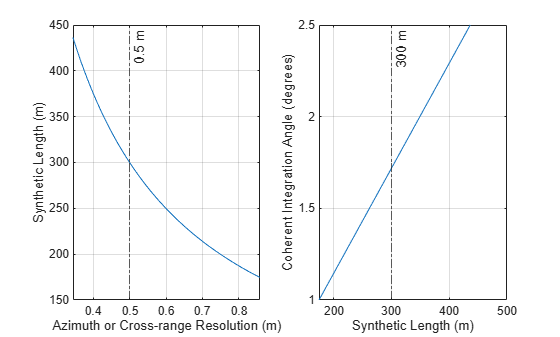The figure indicates that in spotlight SAR mode, a synthetic aperture length of 300 m for the spotlight mode corresponding to an azimuth resolution of 0.5 m. For a coherent integration angle of 1.71 degrees, the azimuth resolution in spotlight mode is 0.5 m. It is important to note that decrease the antenna azimuth dimension to obtain a similar azimuth resolution in stripmap mode.

Set the synthetic aperture length to 300 m and the coherent integration angle to 1.71 degrees for spotlight mode.

`spotlen = 300`
```spotlen = 300 ```
`intang = 1.71`
```intang = 1.7100 ```

Synthetic Beamwidth, Processing Time, and Constraint

Compared to the stripmap mode, spotlight mode can provide a better resolution, and a stronger signal from the scene at the cost of reduced scene size or area imaging rate.

Use the `sarbeamwidth`,` ``sarinttime`,` ``sarmaxcovrate`, and `sarmaxswath` functions to calculate the synthetic beamwidth, integration time, area coverage rate, and maximum swath length. Notice that the area coverage rate and maximum swath length for spotlight SAR system are much smaller than for stripmap mode.

```spotsynbw = sarbeamwidth(lambda,spotlen); % Synthetic beamwidth (degrees) spotinttime = sarinttime(v,spotlen); % Integration time (s) spotcovrate = sarmaxcovrate(spotazres,grazang); % Upper bound on coverage rate (m^2/s) spotswlen = sarmaxswath(v,spotazres,grazang); % Upper bound on swath length (m) SpotlightSAR = [spotsynbw;spotinttime;round(spotcovrate/1e6,1);round(spotswlen/1e3)]; sar = table(Parameters,StripmapSAR,SpotlightSAR,Units)```
```sar=4×4 table Parameters StripmapSAR SpotlightSAR Units ___________________________________ ___________ ____________ _________ "Synthetic Beamwidth" 0.0057294 0.0028628 "degrees" "Integration Time" 1.499 3 "s" "Upper Bound on Swath Length" 173.1 86.5 "km^2/s" "Upper Bound on Area Coverage Rate" 1731 865 "km" ```

### Azimuth Chirp Signal Parameters

Determine the azimuth chirp signal parameters which are the azimuth chirp rate, Doppler bandwidth, beam compression ratio, and azimuth bandwidth after dechirp. You can derive the azimuth time-bandwidth product. These are important for designing an accurate synthetic aperture processing mechanism in azimuth.

Use the `sarchirprate` function to calculate the azimuth chirp rate, which is the rate at which the azimuth signal changes frequency as the sensor illuminates a scatterer.

`azchirp = sarchirprate(maxrng,lambda,v); % (Hz/s)`

Analyze the azimuth chirp rate sensitivity to range and Doppler cone angle variations. The plot shows that increasing the unambiguous range of the radar reduces the azimuth chirp rate.

```dcang = 60:1:120; % Doppler cone angles (in degrees) rngv = 1e3:100:maxrng; azchirpv = zeros(length(dcang),length(rngv)); for i = 1:length(dcang) azchirpv(i,:) = sarchirprate(rngv,lambda,v,dcang(i)); end clf mesh(rngv/1e3,dcang,azchirpv) xlabel('Range (km)') ylabel('Doppler Cone Angle (degrees)') zlabel('Azimuth Chirp Rate (Hz/s)') view([45 45]);```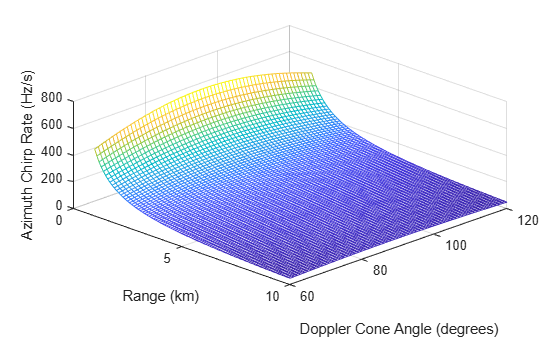Use the `sarscenedopbw` function to calculate the scene bandwidth after azimuth dechirping. Assume a scene size of 916 m.

```Wa = 916; bwdechirp = sarscenedopbw(maxrng,lambda,v,Wa); % (Hz)```

Analyze the scene bandwidth sensitivity to Doppler cone angle variations.

```bwdechirpv = zeros(length(dcang),1); for i = 1:length(dcang) bwdechirpv(i,:) = sarscenedopbw(maxrng,lambda,v,Wa,dcang(i)); end clf plot(dcang,bwdechirpv) grid on xlabel('Doppler Cone Angle (degrees)') ylabel('Azimuth Bandwidth after Dechirp (Hz)')```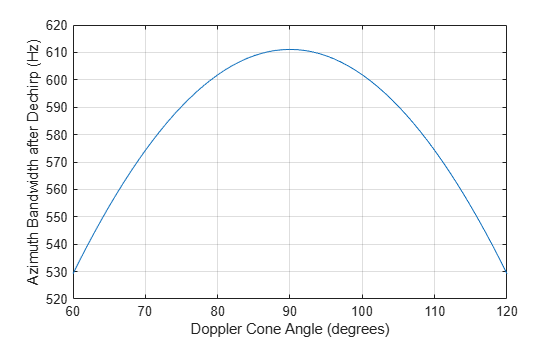Next, use the `sarpointdopbw`` `and` ``sarbeamcompratio` functions to calculate the Doppler bandwidth of the received signal from a point scatterer and the beam compression ratio. Notice that the Doppler bandwidth, and beam compression ratio for a spotlight SAR mode are much greater than for stripmap SAR mode.

```% Stripmap SAR Mode stripbwchirp = sarpointdopbw(v,azres); % (Hz) striptbwaz = bwdechirp*stripinttime; % Unitless stripbcr = sarbeamcompratio(maxrng,lambda,striplen,Wa); % Unitless % Spotlight SAR Mode spotbwchirp = sarpointdopbw(v,spotazres); % (Hz) spottbwaz = bwdechirp*spotinttime; % Unitless spotbcr = sarbeamcompratio(maxrng,lambda,spotlen,Wa); % Unitless Parameters = ["Doppler Bandwidth from Point Scatterer";"Azimuth Time-Bandwidth Product";... "Beam Compression Ratio";"Azimuth Chirp Rate";"Azimuth Bandwidth after Dechirp"]; StripmapSAR = [stripbwchirp;striptbwaz;stripbcr;round(azchirp);bwdechirp]; SpotlightSAR = [spotbwchirp;round(spottbwaz);round(spotbcr);round(azchirp);bwdechirp]; Units = ["Hz";"unitless";"unitless";"Hz/s";"Hz"]; r = table(Parameters,StripmapSAR,SpotlightSAR,Units)```
```r=5×4 table Parameters StripmapSAR SpotlightSAR Units ________________________________________ ___________ ____________ __________ "Doppler Bandwidth from Point Scatterer" 100 200 "Hz" "Azimuth Time-Bandwidth Product" 916.02 1833 "unitless" "Beam Compression Ratio" 916.02 1833 "unitless" "Azimuth Chirp Rate" 67 67 "Hz/s" "Azimuth Bandwidth after Dechirp" 611.09 611.09 "Hz" ```

### SAR Power Calculation

Estimate the peak power that must be transmitted using the power form of the radar equation for stripmap SAR mode. The required peak power depends upon many factors, including the maximum unambiguous range, the required SNR at the receiver, and the pulse width of the waveform. Among these factors, the required SNR at the receiver is determined by the design goal for the Pd and Pfa. Model and estimate the target RCS, the PRF, and different sources of gain and loss for the radar system and its environment.

First, calculate the SNR required at the receiver. The relation between Pd, Pfa, and SNR can be best represented by a receiver operating characteristics (ROC) curve.

```snr_db = [-inf, 0, 3, 10, 13]; rocsnr(snr_db);```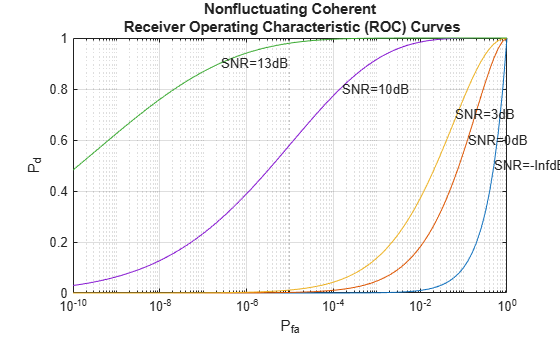The ROC curves show that to satisfy the design goals of Pfa = 1e-6 and Pd = 0.9, the SNR of the received signal must exceed 13 dB. You can surmise the SNR value by looking at the plot, but calculating only the required value is more straightforward. Using the `albersheim` function, derive the required SNR.

`snr_min = albersheim(pd, pfa)`
```snr_min = 13.1145 ```

Target RCS

Use the `landreflectivity` function to calculate the reflectivity, which is the normalized radar cross-section (NRCS) for a given grazing angle and operating frequency. Then calculate the target RCS in the ground image plane using the `sarSurfaceRCS `function and taking the radar resolution into account.

```landType = "Smooth"; nrcs = landreflectivity(landType,grazang,freq); % Calculate normalized RCS of smooth land with no vegetation tgtrcs = sarSurfaceRCS(nrcs,[slantrngres azres],grazang);```

Upper and Lower PRF Bounds

Use the `sarprfbounds` function to determine the minimum and maximum PRF values for a range swath and azimuth resolution given the radar velocity and the grazing angle.

`[prfminv, prfmax] = sarprfbounds(v,azres,rngswath,grazang)`
```prfminv = 100 ```
```prfmax = 6.7268e+04 ```

PRF Selection

The PRF is typically programmable and can be optimized for each application. Use the `sarprf` function to calculate the PRF of the radar based on the radar velocity and the real antenna dimension along the azimuth. Specify a constant roll-off factor as a safety margin to prevent mainlobe returns from aliasing in the PRF interval. If the PRF is set too low, the radar suffers from grating lobes and Doppler ambiguities. If the PRF is set too high, range measurements will be ambiguous.

`prf = sarprf(v,daz,'RollOff',1.5)`
```prf = 150 ```

The selected PRF is within the PRF bounds.

Processing Gains

Use the `matchinggain` function to calculate the range processing gain due to the noise bandwidth reduction after the matched filter.

```d = 0.05; % 5 percent duty cycle pw = (1/prf)*d; % Effective pulse width (s) rnggain = matchinggain(pw,pulse_bw) % Range processing gain (dB)```
```rnggain = 46.9867 ```

Use the `sarazgain` function to calculate the azimuth processing gain due to the coherent integration of pulses.

`azgain = sarazgain(maxrng,lambda,v,azres,prf); % Az processing gain (dB)`

Losses and Noise Factor

Use the `noisefigure` function to estimate the noise figure of the cascaded receiver stages. Assume seven stages with the following values:

• Stage 1 LNA: Noise Figure = 1.0 dB, Gain = 15.0

• Stage 2 RF Filter: Noise Figure = 0.5 dB, Gain = -0.5

• Stage 3 Mixer: Noise Figure = 5.0 dB, Gain = -7.0

• Stage 4 IF Filter: Noise Figure = 1.0 dB, Gain = -1.0

• Stage 5 IF Preamplifier: Noise Figure = 0.6 dB, Gain = 15.0

• Stage 6 IF Stages: Noise Figure = 1.0 dB, Gain = 20.0

• Stage 7 Phase Detectors: Noise Figure = 6.0 dB, Gain = -5.0

```nf = [1.0, 0.5, 5.0, 1.0, 0.6, 1.0, 6.0]; % dB g = [15.0, -0.5, -7.0, -1.0, 15.0, 20.0, -5.0]; % dB cnf = noisefigure(nf, g)```
```cnf = 1.5252 ```

Use the `radarpropfactor` function to calculate the one-way radar propagation factor over smooth land.

```[hgtsd, beta0, vegType] = landroughness('Smooth'); tgtheight = hgtsd; Re = effearthradius(maxrng,h,tgtheight); propf = radarpropfactor(maxrng,freq,h,tgtheight,'EffectiveEarthradius',Re,'TiltAngle',grazang,... 'ElevationBeamwidth',realAntBeamwidth(2),'SurfaceHeightStandardDeviation',hgtsd,'SurfaceSlope',beta0,... 'VegetationType',vegType)```
```propf = -0.0042 ```

Use the `tropopl` function to calculate losses due to atmospheric gaseous absorption.

`atmoLoss = tropopl(maxrng,freq,tgtheight,grazang)`
```atmoLoss = 0.0731 ```

Transmit Power

Use the `radareqsarpow` function to calculate the peak power with the SAR radar equation. You can also specify additional losses and factors, including the azimuth beam shape loss, window loss, transmission loss, and receive line loss. Estimate the beam shape loss with the `beamloss` function, and use 5 dB for all other fixed losses combined. For this analysis, specify `landType` as "`Smooth`" to use the weakest land target. A finite data collection time limits the total energy collected, and signal processing in the radar increases the SNR in the SAR image by two major gain factors. The first is due to pulse compression, and the second is due to coherent integration of pulses.

```imgsnr = snr_min + rnggain + azgain; % (dB) Lb = beamloss; customLoss = 5; % dB sntemp = systemp(cnf); % Noise Temperature Pt = radareqsarpow(maxrng,lambda,imgsnr,pw,rnggain,azgain,'Gain',antGain,'RCS',tgtrcs,... 'AtmosphericLoss',atmoLoss,'Loss',cnf,'PropagationFactor',propf,... 'Ts',sntemp,'CustomFactor',-Lb-customLoss)```
```Pt = 535.0561 ```

### Summary

This example shows the aspects that must be calculated to design an X-band SAR system that can operate in stripmap and spotlight mode. The example shows that the same SAR system can operate in stripmap as well as spotlight modes and achieve varying levels of resolution depending upon the requirements at the cost of other parameters. First, you analyze and select the antenna dimensions to meet the required resolutions. Then you estimate the antenna gains, processing time, constraints, and the azimuth chirp signal parameters. Then estimate the required SNR, target RCS, PRF, processing gains and losses in the radar and its environment. Finally, you use the SAR equation to calculate the peak transmit power.

```Parameters = ["Antenna Dimension in Azimuth";"Antenna Dimension in Elevation";"Synthetic Aperture Length";... "Azimuth Resolution";"Synthetic Beamwidth";"Integration Time";"Upper Bound on Swath Length";... "Upper Bound on Area Coverage Rate";"Coherent Integration Angle";"Doppler Bandwidth from Point Scatterer";... "Azimuth Time-Bandwidth Product";"Beam Compression Ratio";"Azimuth Chirp Rate";"Azimuth Bandwidth after Dechirp"]; Stripmap = [daz;del;striplen;azres;stripsynbw;stripinttime;round(stripcovrate/1e6,1);round(stripswlen/1e3);... NaN;stripbwchirp;striptbwaz;stripbcr;round(azchirp);bwdechirp]; Spotlight = [daz;del;spotlen;spotazres;spotsynbw;spotinttime;round(spotcovrate/1e6,1);round(spotswlen/1e3);... intang;spotbwchirp;round(spottbwaz);round(spotbcr);round(azchirp);bwdechirp]; Units = ["m";"m";"m";"m";"degrees";"s";"km^2/s";"km";"degrees";"Hz";"unitless";... "unitless";"Hz/s";"Hz"]; T = table(Parameters,Stripmap,Spotlight,Units)```
```T=14×4 table Parameters Stripmap Spotlight Units ________________________________________ _________ _________ __________ "Antenna Dimension in Azimuth" 2 2 "m" "Antenna Dimension in Elevation" 0.25 0.25 "m" "Synthetic Aperture Length" 149.9 300 "m" "Azimuth Resolution" 1 0.5 "m" "Synthetic Beamwidth" 0.0057294 0.0028628 "degrees" "Integration Time" 1.499 3 "s" "Upper Bound on Swath Length" 173.1 86.5 "km^2/s" "Upper Bound on Area Coverage Rate" 1731 865 "km" "Coherent Integration Angle" NaN 1.71 "degrees" "Doppler Bandwidth from Point Scatterer" 100 200 "Hz" "Azimuth Time-Bandwidth Product" 916.02 1833 "unitless" "Beam Compression Ratio" 916.02 1833 "unitless" "Azimuth Chirp Rate" 67 67 "Hz/s" "Azimuth Bandwidth after Dechirp" 611.09 611.09 "Hz" ```

### References

 Carrara, Walter G., Ronald M. Majewski, and Ron S. Goodman. Spotlight Synthetic Aperture Radar: Signal Processing Algorithms. Boston: Artech House, 1995.

Supporting Functions

`helperPlotStripmapMode`

```function helperPlotStripmapMode(stripazresv,striplenv,dazv,azres) % Plot azimuth resolution vs. synthetic aperture length subplot(1,2,1) plot(stripazresv,striplenv) grid on xline(azres,'-.',{[num2str(round(azres)),' m']}); % Selected azimuth resolution xlabel('Azimuth or Cross-range Resolution (m)') ylabel('Synthetic Length (m)') stripidx = find(abs(striplenv-150)<1); % Index corresponding to required azimuth resolution % Plot synthetic aperture length vs. antenna azimuth dimensions subplot(1,2,2) plot(striplenv,dazv) grid on xline(striplenv(stripidx),'-.',{[num2str(round(striplenv(stripidx),2)),' m']}); % Selected synthetic length xlabel('Synthetic Length (m)') ylabel('Antenna Azimuth Dimension (m)') end```

`helperPlotSpotlightMode`

```function helperPlotSpotlightMode(spotazresv,spotlenv,intangv,spotazres) % Plot azimuth resolution vs. synthetic aperture length subplot(1,2,1) plot(spotazresv,spotlenv) grid on xline(0.5,'-.',{[num2str(round(spotazres,2)),' m']}); % Selected azimuth resolution xlabel('Azimuth or Cross-range Resolution (m)') ylabel('Synthetic Length (m)') spotidx = find(abs(spotlenv-300)<1); % Index corresponding to 0.5 m azimuth resolution % Plot synthetic aperture length vs. coherent integration angles subplot(1,2,2) plot(spotlenv,intangv) grid on xline(spotlenv(spotidx),'-.',{[num2str(round(spotlenv(spotidx))),' m']}); % Selected synthetic length xlabel('Synthetic Length (m)') ylabel('Coherent Integration Angle (degrees)') end```